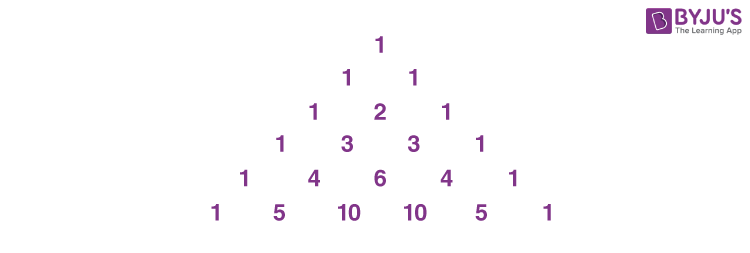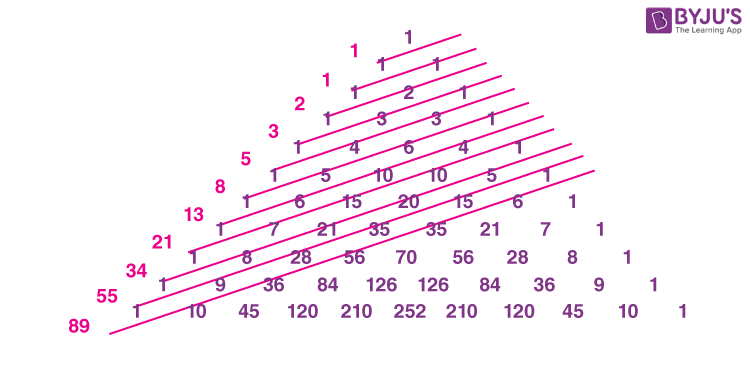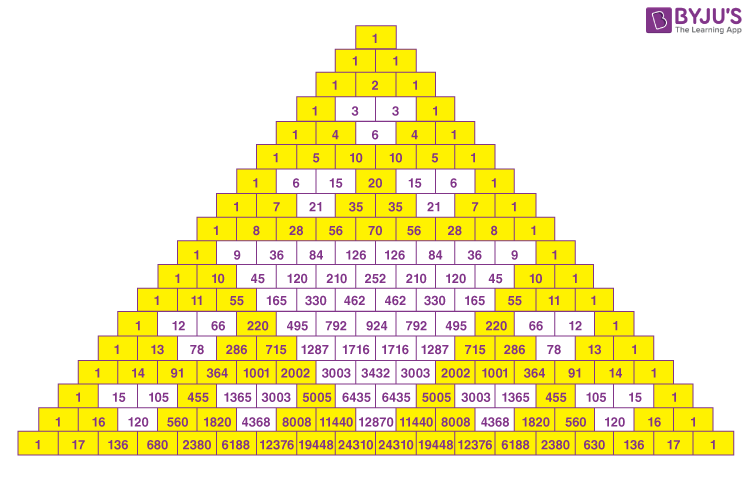# Pascal's Triangle

Pascal’s Triangle is a kind of number pattern. Pascal’s Triangle is the triangular arrangement of numbers that gives the coefficients in the expansion of any binomial expression. The numbers are so arranged that they reflect as a triangle. Firstly, 1 is placed at the top, and then we start putting the numbers in a triangular pattern. The numbers which we get in each step are the addition of the above two numbers. It is similar to the concept of triangular numbersIn this article, we are going to learn Pascal’s triangle history, definition, properties, patterns, formulas and examples with a complete explanation.

## Pascal’s Triangle History

Blaise Pascal was born at Clermont-Ferrand, in the Auvergne region of France on June 19, 1623. In 1653 he wrote the Treatise on the Arithmetical Triangle which today is known as Pascal’s Triangle. Although other mathematicians in Persia and China had independently discovered the triangle in the eleventh century, most of the properties and applications of the triangle were discovered by Pascal.

This triangle was among many of Pascal’s contributions to mathematics. He also came up with significant theorems in geometry, discovered the foundations of probability and calculus and also invented the Pascaline-calculator. Still, he is best known for his contributions to the Pascal triangle.

## Pascal’s Triangle Definition

Most people are introduced to Pascal’s triangle through an arbitrary-seeming set of rules. Begin with 1 on the top and with 1’s running down the two sides of a triangle. Each new number lies between two numbers and below them, and its value is the sum of the two numbers above it. The theoretical triangle is infinite and continues downward forever, but only the first 6 lines appear in figure 1. More rows of Pascal’s triangle are listed in the last figure of this article. A different way to describe the triangle is to view the first line is an infinite sequence of zeros except for a single 1. To obtain successive lines, add every adjacent pair of numbers and write the sum between and below them. The non-zero part is Pascal’s triangle.

## Pascal’s Triangle Construction

The easiest way to construct the triangle is to start at row zero and write only the number one. From there, to obtain the numbers in the following rows, add the number directly above and to the left of the number with the number above and to the right of it. If there are no numbers on the left or right side, replace a zero for that missing number and proceed with the addition. Here is an illustration of rows zero to five.From the above figure, if we see diagonally, the first diagonal line is the list of ones, the second line is the list of counting numbers, the third diagonal is the list of triangular numbers and so on.

## Pascal’s Triangle Formula

The formula to find the entry of an element in the nth row and kth column of a pascal’s triangle is given by:

$$\begin{array}{l}i.e.,{n \choose k}\end{array}$$

The elements of the following rows and columns can be found using the formula given below:

$$\begin{array}{l}Pascal’s\ Triangle\ Formula = {n \choose k}= {n-1 \choose k-1}+ {n-1 \choose k}\end{array}$$

Here, n is any non-negative integer and 0 ≤ k ≤ n.

The above notation can be written as:

$$\begin{array}{l}{n \choose k} (i.e., n\ choose\ k) = C(n, k) = \ ^{n}C_{k} = \frac{n!}{[k!(n-k)!]}\end{array}$$

This pattern of getting binomial coefficients is called Pascal’s rule.

## Pascal’s Triangle Binomial Expansion

Pascal’s triangle defines the coefficients which appear in binomial expansions. That means the nth row of Pascal’s triangle comprises the coefficients of the expanded expression of the polynomial (x + y)n.

The expansion of (x + y)n is:

(x + y)n = a0xn + a1xn-1y + a2xn-2y2 + … + an-1xyn-1 + anyn

where the coefficients of the form ak are precisely the numbers in the nth row of Pascal’s triangle. This can be expressed as:

$$\begin{array}{l}a_{k}= {n \choose k}\end{array}$$

For example, let us expand the expression (x + y)n for n = 3.

(x + y)3 = 3C0x3 + 3C1 x2y + 3C2 xy2 + 3C3 x0y3

= (1)x3 + (3)x2y + (3)xy2 + (1)y3

Here, the coefficients 1, 3, 3, 1 represent elements in the 3rd row of the pascal’s triangle.

## How to Use Pascal’s Triangle?

Pascal’s triangle can be used in various probability conditions. Suppose if we are tossing the coin one time, then there are only two possibilities of getting outcomes, either Head (H) or Tail (T).

If we toss it two times, then there are one possibility of getting both heads HH and both as tails TT, but there are two possibilities of getting at least a Head or a Tail, i.e. HT or TH.

Now you may consider how Pascal’s triangle will help here. So let’s see the table given here based on the number of tosses and outcomes.

 Number of Tosses Number of Outcomes Pascal’s Triangle 1 H T 1,1 2 HH HT TH TT 1, 2, 1 3 HHH HHT, HTH, THH HTT, THT, TTH TTT 1,3,3,1

We can also extend it by increasing the number of tosses.

## Pascal’s Triangle Patterns

One of the interesting properties of the triangle is that the sum of numbers in a row is equal to 2n

where n corresponds to the number of the row:

1 = 1 = 20

1 + 1 = 2 = 21

1 + 2 + 1 = 4 = 22

1 + 3 + 3 + 1 = 8 = 23

1 + 4 + 6 + 4 + 1 = 16 = 24

### Prime Numbers in the Triangle

Another pattern visible in the triangle deals with prime numbers. If a row starts with a prime number or is a prime numbered row, all the numbers that are in that row (not counting the 1’s) are divisible by that prime. If we look at row 5 (1 5 10 10 51), we can see that 5 and 10 are divisible by 5. However, for a composite numbered row, such as row 8 (1 8 28 56 70 56 28 8 1), 28 and 70 are not divisible by 8.

### Fibonacci Sequence in the Triangle

By adding the numbers in the diagonals of the Pascal triangle the Fibonacci sequence can be obtained as seen in the figure given below.There are various ways to show the Fibonacci numbers on the Pascal triangle. R. Knott was able to find the Fibonacci appearing as sums of “rows” in the Pascal triangle. He moved all the rows over by one place and here the sums of the columns would represent the Fibonacci numbers.

## Pascal’s Triangle Properties

• Each number is the sum of the two numbers above it.
• The outside numbers are all 1.
• The triangle is symmetric.
• The first diagonal shows the counting numbers.
• The sums of the rows give the powers of 2.
• Each row gives the digits of the powers of 11.
• Each entry is an appropriate “choose number.”
• And those are the “binomial coefficients.”
• The Fibonacci numbers are there along diagonals.

Here is an 18-lined version of pascal’s triangle;## Video Lesson

### Pascals Triangle### Pascal’s Triangle Examples

Example 1:

Find the third element in the fourth row of Pascal’s triangle.

Solution:

To find: 3rd element in 4th row of Pascal’s triangle.

As we know that the nth row of Pascal’s triangle is given as nC0, nC1, nC2, nC3, and so on.

Thus, the formula for Pascal’s triangle is given by:

nCk = n-1Ck-1  + n-1Ck

Here, nCrepresnts (k+1)th element in the nth row.

Now, to determine the 3rd element in the 4th row, we have to calculate 4C2.

Therefore, 4C= 4-1C2-1 + 4-1C2

4C= 3C1 + 3C2

4C= 3 + 3  [Since 3C= 3, 3C= 3]

4C= 6.

Therefore, the third element in the fourth row of Pascal’s triangle is 6.

Example 2:

Determine the coefficients of expansions of (x+y)2 using Pascal’s triangle.

Solution:

As we know that the coefficients of expansion of (x+y)should be the elements in the second row of Pascal’s triangle.

Since the elements in the 2nd row of Pascal’s triangle are 1, 2, 1, the coefficients of the expansion of (x+y)should be 1, 2, 1.

### Practice Questions on Pascal’s Triangle

Solve the following problems based on Pascal’s triangle:

1. Determine the fifth element in the 6th row of Pascal’s triangle using Pascal’s formula.
2. What is the sum of elements in the 10th row of Pascal’s triangle?
3. Find the coefficients of the expansions of  (a+b)using Pascal’s triangle.

## Frequently Asked Questions on Pascal’s Triangle

Q1

### What is Pascal’s Triangle?

Pascal’s triangle is the triangular array of numbers that begins with 1 on the top and with 1’s running down the two sides of a triangle. Each new number lies between two numbers and below them, and its value is the sum of the two numbers above it.

Q2

### What are the applications of Pascal’s Triangle?

Pascal’s triangle has various applications in Mathematics, such as in algebra, probability theory, combinatorics, statistics, and so on. Pascal’s triangle can be used to calculate the combinations.

Q3

### What are the patterns found in Pascal’s Triangle?

The patterns found in Pascal’s triangle are:
Triangular Pattern
Odd and even pattern
Fibonacci pattern
Symmetrical pattern

Q4

### What is the 5th row of Pascal’s triangle?

The fifth row of Pascal’s triangle is 1 5 10 10 5 1. The sum of the elements in the fifth row of Pascal’s triangle is 32, which can be verified using the formula, 2n. (i.e) 2n = 32.

Q5

### Does Pascal’s triangle have a symmetric pattern?

Yes, Pascal’s triangle has a symmetric pattern. The numbers on the left side of the triangle have identical matching numbers on the right side. Hence, we can say that Pascal’s triangle is symmetrical.